# RD Sharma Solutions Class 12 Algebra Of Vectors Exercise 23.2

RD Sharma Solutions for Class 12 Maths Chapter 23 Algebra of Vectors Exercise 23.2 are enclosed here. Top subject-matter experts at BYJU’S have designed these solutions in a way that is easily understood by every student. Moreover, all the solutions are written in a simple stepwise format.  Here, the RD Sharma Class 12 Solutions to this exercise are contained in PDF format, which can be downloaded easily from the link given below.

## Download PDF of Rd Sharma Solution for Class 12 Maths Chapter 23 Exercise 2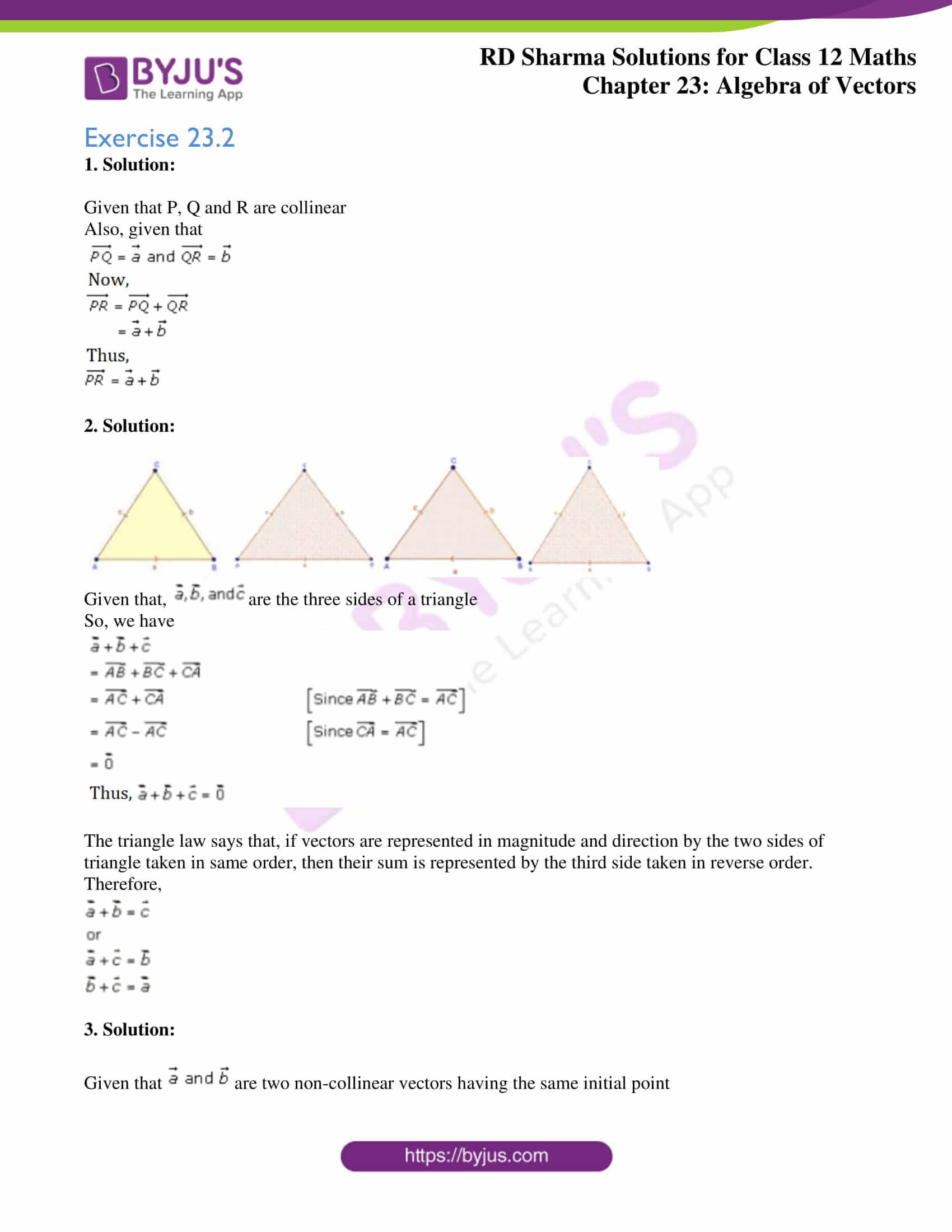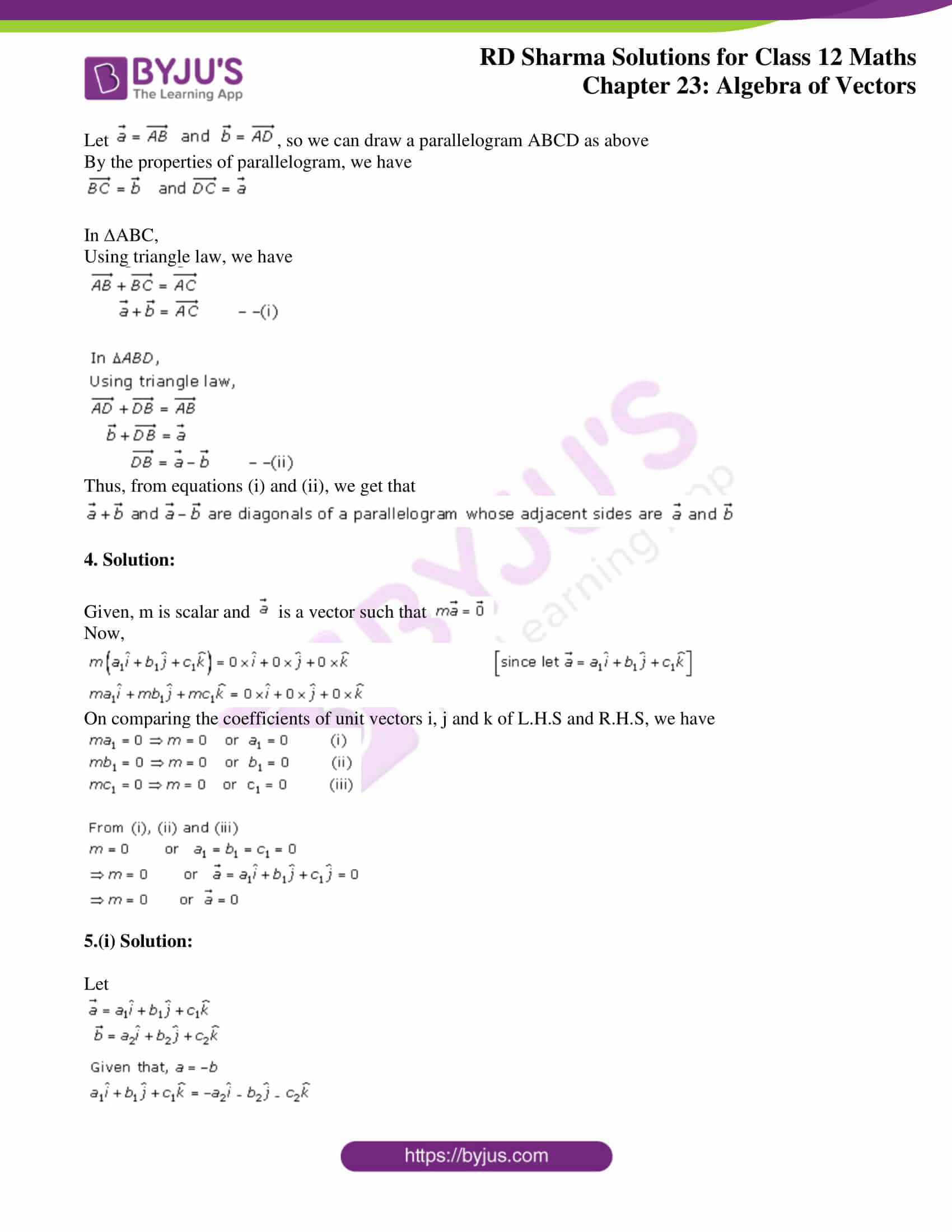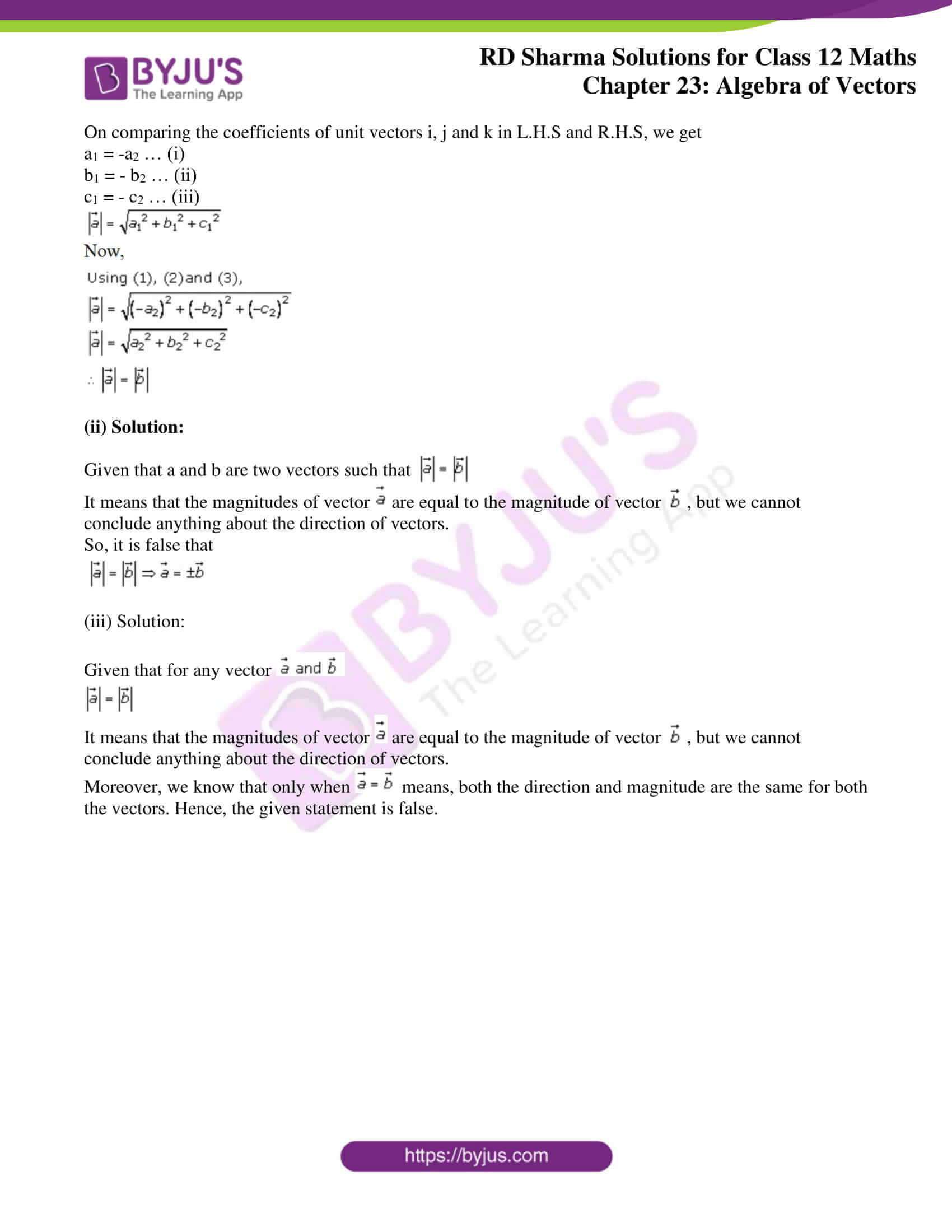### Access Answers for Rd Sharma Solution Class 12 Maths Chapter 23 Exercise 2

Exercise 23.2

1. Solution:

Given that P, Q and R are collinear

Also, given that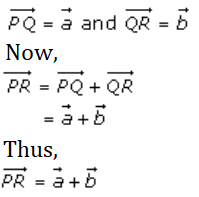2. Solution: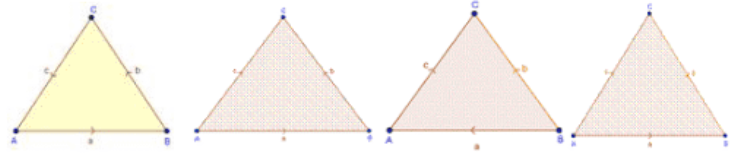Given that,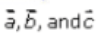are the three sides of a triangle

So, we have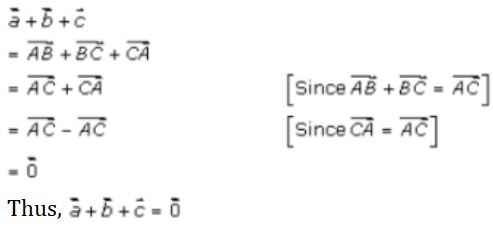The triangle law says that, if vectors are represented in magnitude and direction by the two sides of triangle taken in same order, then their sum is represented by the third side taken in reverse order.

Therefore,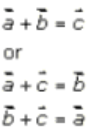3. Solution:

Given that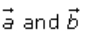are two non-collinear vectors having the same initial point

Let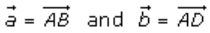, so we can draw a parallelogram ABCD as above

By the properties of parallelogram, we have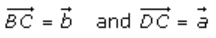In ∆ABC,

Using triangle law, we have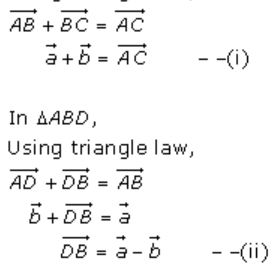Thus, from equations (i) and (ii), we get that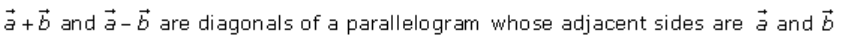4. Solution:

Given, m is scalar and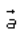is a vector such that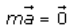Now,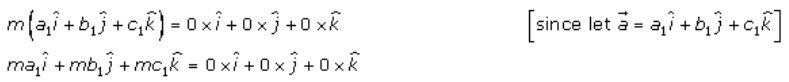On comparing the coefficients of unit vectors i, j and k of L.H.S and R.H.S, we have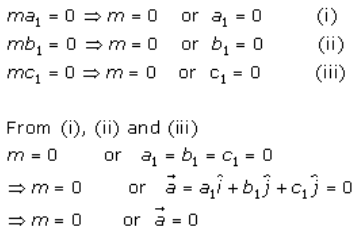5.(i) Solution:

Let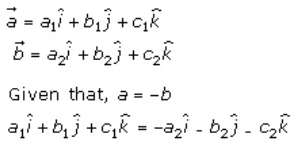On comparing the coefficients of unit vectors i, j and k in L.H.S and R.H.S, we get

a1 = -a2 … (i)

b1 = – b2 … (ii)

c1 = – c2 … (iii)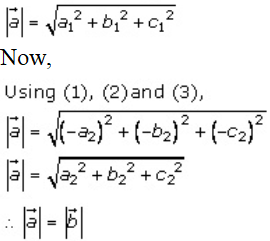(ii) Solution: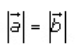Given that a and b are two vectors such that

It means that the magnitudes of vector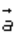are equal to the magnitude of vector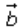, but we cannot conclude anything about the direction of vectors.

So, it is false that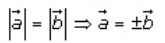(iii) Solution:

Given that for any vector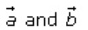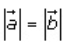It means that the magnitudes of vectorare equal to the magnitude of vector, but we cannot conclude anything about the direction of vectors.

Moreover, we know that only when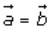means, both the direction and magnitude are the same for both the vectors. Hence, the given statement is false.